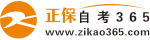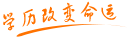#24小时客服：010-82335555# 2003年4月全国高等教育自学考试计算机软件基础（一）试题

2005年06月11日    来源：   字体：   打印

课程代码：02243

第一部分   选择题   （共40分）

一、单项选择题（本大题共40小题，每小题1分，共40分）

在每小题列出的四个备选项中只有一个是符合题目要求的，请将其代码填写在题后的括号内。错选、多选或未选均无分。

1.C语言正确的类型说明符是（   ）

A.Char   B.char

C.real   D.string

2.C语言中，已知：int i，j，k；下列运算符使用错误的是（   ）

A.i++   B.j-

C.++k   D.8++

3.下列叙述中错误的描述是（   ）

A.一个C源程序至少包括一个函数。

B.一个C源程序可以包含一个main（）函数和其它函数。

C.主函数和其它函数可以互相调用。

D.C程序的基本单位是函数。

4.C语言中，字符串在计算机中的结束标志是（   ）

A.空格   B.换行

C.′\0′   D.′\t′

5.C语言中，s=i++；等价于（   ）

A.s=i；i=i+1；   B.i=i+1；s=i；

C.s=i；s=s+1；   D.s=i；s=i+1；

6.C语言中，赋值语句的赋值号（“=”）左边（   ）

A.可以是常数   B.可以是变量名

C.只能是表达式   D.可以是常数或表达式

7.下列程序的运行结果是（   ）

#include <stdio.h>

#include <math.h>

main（）

{ int a=3；

a=3+a%4+sqrt（9）；

a=a/3；

a++；

printf（″%d\n″，a）；

}

A.2   B.2.6

C.3   D.4

8.下列程序的运行结果是（   ）

#include <stdio.h>

main（）

{ int a=15；

printf（″%d\n″，（a>10   40：50））；

}

A.10   B.15

C.40   D.50

9.代数式 ，其错误的C语言表达式是（   ）

A.a*d/b/c*3   B.3*a*d/b*c

C.a/b*d/c*3   D.3*a*d/b/c

10.已知：int u=010，v=0x10，w=10；则printf（″%d，%d，%d\n″，u，v，w）；执行后的输出结果是（   ）

A.8，8，10   B.8，10，10

C.8，16，10   D.10，10，10

11.C语言中，下列运算符优先级最高的是（   ）

A.>=   B.==

C.！=   D.&&

12.C语言中，if和switch语句属于（   ）

A.说明语句   B.注释语句

C.程序流程控制语句   D.顺序执行语句

13.已知：int a=1，b=2，c=3，d=4；则表达式a>b   a：（c>d   c：d）的值是（   ）

A.1   B.2

C.3   D.4

14.下列程序段的输出结果是（   ）

int a=2，b=3，c=4，e=5；

e=a+b>c && b==c；

printf（″e=%d″，e）

A.e=0   B.e=1

C.e=4   D.e=5

15.C语言中，语句while后一对圆括号中的表达式（   ）

A.只能是逻辑表达式

B.只能是关系表达式

C.只能是关系表达式或逻辑表达式

D.可以是任意表达式

16.C语言中，下列只循环一次的do-while循环是（   ）

A.do   B.do

{；   {；

} while（5）；   }while（k=0）；

C.do   D.int k=5；

{；   do

} while（-1）；   {k-；

}while（k）；

17.C语言程序中，for循环语句中的表达式2为一非零常数且循环体内无break语句及goto语句，则循环体的执行次数为（   ）

A.0次   B.表达式2表示的非零常数

C.不确定，由循环体决定   D.无穷次

18.下列程序的运行结果是（   ）

#include <stdio.h>

mian（）

{ int x=3，y=6，z=0；

while（x++！=（y=y-1））

{ z++；

if（y<x） break；

}

printf （“x=%d，y=%d，z=%d”，x，y，z）；

}

A.x=4，y=4，z=1   B.x=5，y=4，z=1

C.x=5，y=4，z=2   D.x=5，y=5，z=1

19.C语言中，下列定义数组的正确语句是（   ）

A.char a，b；   B.char a&#；；

C.int b[″7″]；   D.int j=3；int a[j]；

20.已知：char a&#；={′0   ′， ′0   ′， ′0   ′， ′0   ′， ′0   ′， ′0   ′， ′0   ′， ′0   ′}；则（   ）

A.该数组元素个数为7   B.该数组长度不确定

C.该数组元素的最大下标是7   D.该数组至少有9个数组元素

21.已知：char str&#；=″\t\v\56\7 morning″；则strlen（str）的值是（   ）

A.8   B.11

C.16   D.17

22.已知：char a&#；=″abcdef″；char b&#；={′a′，′b′，′c′，′d′，′e′，′f′}；则（   ）

A.a数组的长度比b数组长   B.a数组和b数组长度相等

C.a数组的长度比b数组短   D.a数组和b数组完全相同

23.下列程序的运行结果是（   ）

#include <stdio.h>

int mul（int x）

{int z；

z=x*x；

return z；

}

main（）

{int a=10，k=2，m=1；

a=a/（mul（k+m）/mul（k+m））；

printf（″%d\n″，a）；

}

A.0   B.1

C.9   D.10

24.关于C语言函数，正确的叙述是（   ）

A.实参与形参的类型应一致，当形参为整型时，实参也必须为整型。

B.函数可以用void定义为“无类型”，函数不返回值。

C.函数如有return语句，则return语句中表达式的类型必须和函数类型一致。

D.函数类型为实型时，类型标识符可以省略。

25.能正确定义指针变量cp的C语言语句是（   ）

A.char *cp=&′0′；   B.char cp；

C.char cp=″abc″；   D.char ch，*cp=&ch；

26.指针变量作为形参时，下列错误的叙述是（   ）

A.实参可以为同类型指针变量。

B.形参的变化将改变相应实参的地址。

C.实参可以为同类型数组变量。

D.可以实现函数间多个数据的传递。

27.已知：int a，*ip；使ip指向a的正确语句是（   ）

A.ip=&a；   B.*ip=a；

C.*ip=&a；   D.ip=a；

28.已知：char *cp=″12345″；则printf（″%s″，++cp）；的输出结果是（   ）

A.1   B.2

C.2345   D.12345

29.如果磁盘上没有文本文件test.dat，执行下列程序段后，其结果是（   ）

FILE *fp；

fp=fopen（″test.dat″，″r″）；

A.系统打开文件test.dat，且fp的值不是NULL.

B.系统建立新文件test.dat.

C.fp的值是NULL.

D.fp的值不确定。

30.对文本文件正确的叙述是（   ）

A.文本文件占用存储空间较少，但不能直接在屏幕上原样显示。

B.文本文件可以在屏幕上原样显示，但占用存储空间较大。

C.文本文件占用存储空间大且不能直接在屏幕上原样显示。

D.文本文件占用存储空间较小且可以直接在屏幕上原样显示。

31.FoxPro中，内存变量文件的扩展名是（   ）

A.DBF   B.IDX

C.BAK   D.MEM

32.FoxPro中，命令“TOTAL ON 单位 TO XM”中的XM是（   ）

A.内存变量名   B.字段变量名

C.数据库文件名   D.文本文件名

33.FoxPro中，已知内存变量A=″Fox □″，B=″Pro″，则函数LEN（A-B）的值是（注：□表示空格）（   ）

A.3   B.4

C.6   D.7

34.FoxPro中，执行命令FNAME=″ST″和USE &FNAME后，打开的数据库文件是（   ）

A.&FNAME.DBF   B.FNAME.DBF

C.ST.DBF   D.ST.IDX

35.现有P1，P2两个过程，要根据N（1或2）值的不同调用相应的程序，下列四个程序段中会出现错误的程序段是（   ）

A.CLEAR   B.INPUT TO N

SET TALK OFF   DO CASE

ACCEPT TO N   CASE N=1

H=′P′+N   DO P1

DO H   OTHERWISE

SET TALK ON   DO P2

RETURN   ENDCASE

C.INPUT TO N   D. ACCEPT TO N

IF N=1   DO CASE

DO P1   CASE N=′1′

ELSE   DO P1

DO P2   CASE N=′2′

ENDIF   DO P2

ENDCASE

36.已知学生记录描述为：

struct student

{ int no；

char name，sex；

struct

{ int year，month，day；

} birth；

}；

struct student s；

设变量s中的“生日”是“1984年11月12日”，对“birth”正确赋值的程序段是（   ）

A.year=1984；month=11；day=12；

B.s.year=1984；s.month=11；s.day=12；

C.birth.year=1984；birth.month=11；birth.day=12；

D.s.birth.year=1984；s.birth.month=11；s.birth.day=12；

37.已知下列定义语句，则对pup中成员sex的正确引用是（   ）

struct pupil

{ char name；

int sex；

} pup，*p=&pup；

A.p.pup.sex   B.p->pup.sex

C.（*p）。pup.sex   D.（*p）。sex

38.入队运算（   ）

A.只能在队头进行。   B.只能在队尾进行。

C.可以在队头或队尾进行。   D.可以在队列中间的任意位置进行。

39.假定用上界为m的向量s（1∶m）存储栈，设栈顶指针top总是指向栈顶元素，要将x入栈的操作步骤是（   ）

A.top=top+1；s[top]=x；   B.s[top]=x；top=top+1；

C.top=（top+1）%m；s[top]=x；   D.s[top]=x；top=（top+1）%m；

40.将递归算法转化为非递归算法通常使用的数据结构是（   ）

A.栈   B.队列

C.链表   D.数组

第二部分   非选择题   （共60分）

二、填空题（本大题共10个空，每空1分，共10分）

请在每小题的空格中填上正确答案。错填、不填均无分。

41.C语言中，唯一的三目运算符是_______，而&&是_______目运算符。

42.执行下列程序段后，i的值是_______，j的值是_______.

int i，j=5；

for（i=0；i<5；i++）

j=j+i；

43.下列程序的功能是将字符串″country″纵向显示在屏幕上。填空完成程序。

#include<stdio.h>

main（）

{ static char a&#；=″country″；

int i；

for（i=0；a[i]！=′\0′；_______）

printf（″%c\n″，_______）；

}

44.FoxPro支持三种工作方式，它们是_______工作方式、_______工作方式和菜单工作方式。

45.C语言中，要动态申请内存空间可使用函数_______；要释放动态空间可使用函数_______.

三、阅读程序题（本大题共8小题，每小题4分，共32分）

46.写出下列程序的运行结果。

#include <stdio.h>

main（）

{ int a，b=0，m=3，n=2；

a=（m>5） && （（b=3）>n）；

printf（″a=%d，b=%d″，a，b）；

}

结果：

47.下列程序的功能是输出1至100之间的自然数中各位数字的乘积大于各位数字之和的那些数，填空完成程序。（例如：58，各位数字的乘积为5*8=40，各位数字之和为5+8=13）

#include <stdio.h>

main（）

{ int n，k，s，m；

for （n=1；n<=100；n++）

{ k=1；s=0；

m=n；

while （_______）

{ k*=m%10；

s+=m%10；

m=m/10；

}

if （_______） printf（″%d″，n）；

} }

48.下列程序的功能是：从键盘输入一个数值插入有序数组，插入后仍保持数组元素值有序。填空完成程序。

#include <stdio.h>

main（）

{ int a ={2，4，6，8，10，12，14，16，18，20}，i，x；

scanf（″%d″，&x）；

for （i=9；i>=0；i-）

{ if （a[i]>x）

a[i+1]=a[i]；

else

{ a[i+1]=x；

_______；

}

}

if（_______）a